# Create Date Range from Two Dates – Excel & Google Sheets

This tutorial will demonstrate how to create a date range from two dates.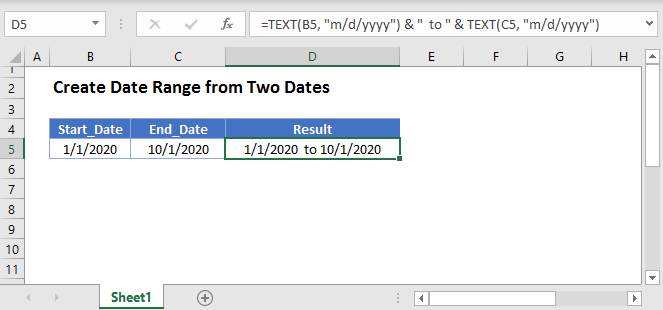## Date as Text

The first step to creating a date range from two dates is to convert a date to text using the TEXT Function. The TEXT Function can convert dates into many different formats, but we’ll focus on two.

### M/D/YYYY Format

``=TEXT(B3,"M/D/YYYY")``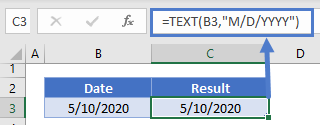### MMMM D, YYYY Format

``=TEXT(B3,"mmmm d, yyyy")``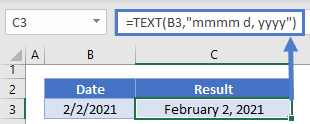## Create Date Range

We can concatenate (join) two dates stored as text using the & operator:

``=TEXT(B3, "m/d/yyyy") & "  to " & TEXT(C3, "m/d/yyyy")``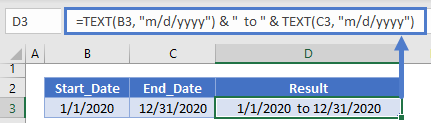This is useful for an event start and end date, but what if the second date is missing (ex. the event is only one day):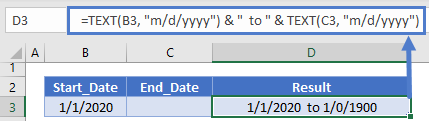To avoid the erroneous ” to ” if the second date is missing, we can use an If Statement to test if the second date exists before adding it to the string:

``=TEXT(B3, "m/d/yyyy") & IF(C3<>"", " to " & TEXT(C3, "m/d/yyyy"), "")``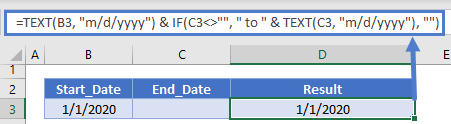You can also handle if the first date is missing:

``=IF(B3<>"",TEXT(B3, "m/d/yyyy")&" to " &TEXT(C3, "m/d/yyyy"),TEXT(C3,"m/d/yyyy") )``

## Create Date Range from Two Dates in Google Sheets

All of the above examples work exactly the same in Google Sheets as in Excel.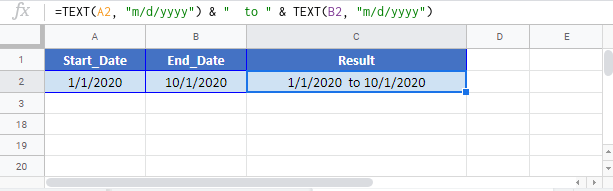### Excel Practice Worksheet

Practice Excel functions and formulas with our 100% free practice worksheets!